### Home > MC1 > Chapter 7 > Lesson 7.2.4 > Problem7-81

7-81.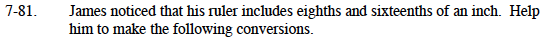You may have noticed that 8 is half of 16.

$\text{ It will help to know that it is also true that the number of }\frac{1}{8}\text{'s is equal to half the number of }\frac{1}{16}\text{'s}.$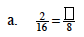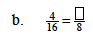$\text{Remember that the number of }\frac{1}{8}\text{'s is half the number of } \frac{1}{16}\text{'s. What is half of four?}$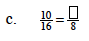5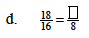9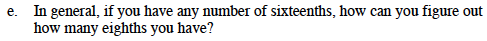How were you able to solve parts (a) through (d)?